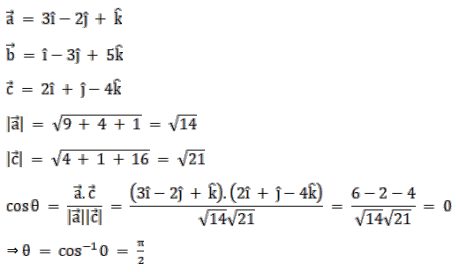# Solve this followingQuestion:

Show that the vectors $\vec{a}=(3 \hat{i}-2 \hat{j}+\hat{k}), \vec{b}=(\hat{i}-3 \hat{j}+5 \hat{k})$ and $\vec{c}=(2 \hat{i}+\hat{j}-4 \hat{k})$ form a right - angled triangle.

Solution:Hence, the triangle is a right angled triangle at c